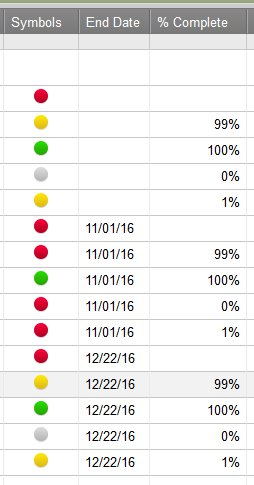#### Welcome to the Smartsheet Forum Archives

The posts in this forum are no longer monitored for accuracy and their content may no longer be current. If there's a discussion here that interests you and you'd like to find (or create) a more current version, please Visit the Current Forums.

# nested forumlas

Hello,

I'm having trouble with my nested formula and activating the Red, Green, Gray, Yellow ball based on the % compelte column.

=IF([% Complete] = IS BLANK, activate the RED ball

=IF([% Complete] = less than 100%, activate the YELLOW ball

=IF([% Complete] = 100% activate the GREEN ball

=IF([% Complete] = 0% activate the GRAY ball

Addtionally, if it is past the deadline and not green activated can it automatically activiate the Red flag symbol?

Tags:

• Here you go:

=IF([% Complete]2 = 1, "Green", IF(AND(ISDATE([End Date]2), [End Date]2 < TODAY()), "Red", IF(ISBLANK([% Complete]2), "Red", IF([% Complete]2 = 0, "Gray", IF([% Complete]2 > 0, "Yellow", "other")))))

or formatted for legibility:

=IF([% Complete]2 = 1, "Green",

IF(AND(ISDATE([End Date]2), [End Date]2 < TODAY()), "Red",

IF(ISBLANK([% Complete]2), "Red",

IF([% Complete]2 = 0, "Gray", IF([% Complete]2 > 0, "Yellow", "other")))))

Here's the results:

Tips for nested If's:

IF's are processed left to right - when the result is true, the false branch is not checked or processed (and the reverse is true).

Watch out for satisfying the check before you intend to -- like checking for less than 100% and then checking for 0%. 0 is less than 100 so it won't get there.

(That's why we check for gray before we check for yellow, and why we check for a past due date before we care about what value other than 100% it might be)

Enjoy

CraigThis discussion has been closed.